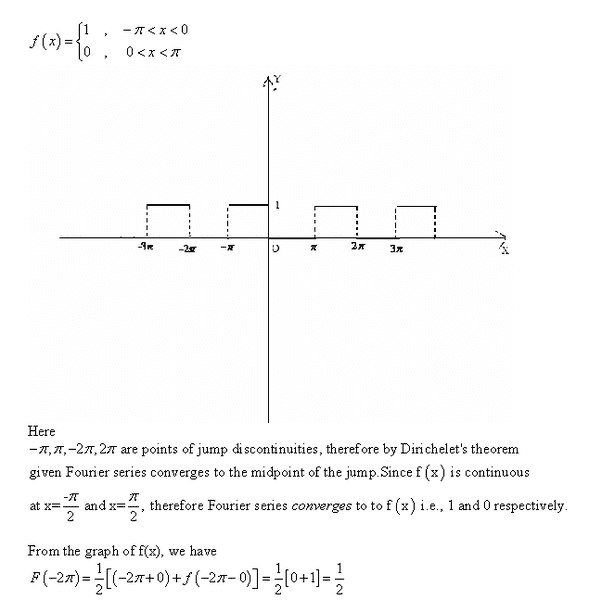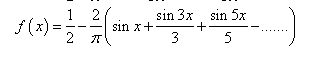# Fourier series convergence

## Homework StatementThis series is what dictates the graph above.## The Attempt at a Solution

I don't understand what's going on. If they're using the series that i pasted below then why aren't they multiply each value in the brackets by -2/pi?

I also don't get why terms in the brackets are

[(-2pi + 0) + f(-2pi - 0)]

Where did they come from? Where does 1/2 come from that multiplies the brackets. very confused.

LCKurtz
Homework Helper
Gold Member
I don't understand what's going on. If they're using the series that i pasted below then why aren't they multiply each value in the brackets by -2/pi?

They could have. The ##\frac {-2}\pi## was just factored out.
I also don't get why terms in the brackets are

[(-2pi + 0) + f(-2pi - 0)]

Where did they come from? Where does 1/2 come from that multiplies the brackets. very confused.

Your function isn't defined at the jumps, like at ##-2\pi##. To get the average value at the jumps you need the average of the right and left limits:$$\lim_{h\rightarrow 0+}\frac{f(-2\pi + h)+f(-2\pi -h)}{2}$$Those limits give the value of the function at ##-2\pi\ ##"coming from" the left and right. The expression ##f(-2\pi + 0)+ f(-2\pi -0)##is just a shorthand notation for the limits, and you add them and divide by 2 to get the average.